CBSE (Science) Class 11CBSE
Share

# H.C. Verma solutions for Class 12 Physics chapter 4 - Laws of Thermodynamics

## Concepts of Physics - Vol. 2

#### H.C. Verma Concepts of Physics - Vol. 2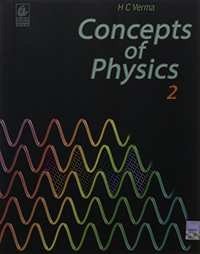## Chapter 4: Laws of Thermodynamics

#### Chapter 4: Laws of Thermodynamics Exercise Short Answers solutions [Page 60]

Short Answers | Q 1 | Page 60

Should the internal energy of a system necessarily increase if heat is added to it?

Short Answers | Q 2 | Page 60

Should the internal energy of a system necessarily increase if its temperature is increased?

Short Answers | Q 3 | Page 60

A cylinder containing a gas is lifted from the first floor to the second floor. What is the amount of work done on the gas? What is the amount of work done by the gas? Is the internal energy of the gas increased? Is the temperature of the gas increased?

Short Answers | Q 4 | Page 60

A force F is applied on a block of mass M. The block is displaced through a distance d in the direction of the force. What is the work done by the force on the block? Does the internal energy change because of this work?

Short Answers | Q 5 | Page 60

The outer surface of a cylinder containing a gas is rubbed vigorously by a polishing machine. The cylinder and its gas become warm. Is the energy transferred to the gas heat or work?

Short Answers | Q 6 | Page 60

When we rub our hands they become warm. Have we supplied heat to the hands?

Short Answers | Q 7 | Page 60

A closed bottle contains some liquid. the bottle is shaken vigorously for 5 minutes. It is found that the temperature of the liquid is increased. Is heat transferred to the liquid? Is work done on the liquid? Neglect expansion on heating.

Short Answers | Q 8 | Page 60

The final volume of a system is equal to the initial volume in a certain process. Is the work done by the system necessarily zero? Is it necessarily nonzero?

Short Answers | Q 9 | Page 60

Can work be done by a system without changing its volume?

Short Answers | Q 10 | Page 60

An ideal gas is pumped into a rigid container having diathermic walls so that the temperature remains constant. In a certain time interval, the pressure in the container is doubled. Is the internal energy of the contents of the container also doubled in the interval ?

Short Answers | Q 11 | Page 60

When a tyre bursts, the air coming out is cooler than the surrounding air. Explain.

Short Answers | Q 12 | Page 60

When we heat an object, it expands. Is work done by the object in this process? Is heat given to the object equal to the increase in its internal energy?

Short Answers | Q 13 | Page 60

When we stir a liquid vigorously, it becomes warm. Is it a reversible process?

Short Answers | Q 14 | Page 60

What should be the condition for the efficiency of a Carnot engine to be equal to 1?

Short Answers | Q 15 | Page 60

When an object cools down, heat is withdrawn from it. Does the entropy of the object decrease in this process? If yes, is it a violation of the second law of thermodynamics stated in terms of increase in entropy?

#### Chapter 4: Laws of Thermodynamics Exercise MCQ solutions [Page 61]

MCQ | Q 1 | Page 61

The first law of thermodynamics is a statement of ____________ .

• conservation of heat

• conservation of work

• conservation of momentum

• conservation of energy

MCQ | Q 2 | Page 61

If heat is supplied to an ideal gas in an isothermal process, _____________ .

• the internal energy of the gas will increase

• the gas will do positive work

• the gas will do negative work

• the said process is not possible

MCQ | Q 3 | Page 61

Figure shows two processes A and B on a system. Let ∆Q1 and ∆Q2 be the heat given to the system in processes A and B respectively. Then ____________ .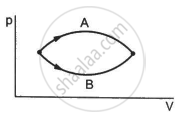• ∆Q1 > ∆Q2

• ∆Q1 = ∆Q2

• ∆Q1 < ∆Q2

• ∆Q1 ≤ ∆Q2

MCQ | Q 4 | Page 61

Refer to figure. Let ∆U1 and ∆U2 be the changes in internal energy of the system in the process A and B. Then _____________ .• ∆U1 > ∆U2

• ∆U1 = ∆U2

• ∆U1 < ∆U2

• ∆U1 ≠ ∆U2

MCQ | Q 5 | Page 61

Consider the process on a system shown in figure. During the process, the work done by the system ______________ .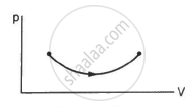• continuously increases

• continuously decreases

• first increases then decreases

• first decreases then increases

MCQ | Q 6 | Page 61

Consider the following two statements.

(A) If heat is added to a system, its temperature must increase.

(B) If positive work is done by a system in a thermodynamic process, its volume must increase.

• Both A and B are correct

• A is correct but B is wrong

• B is correct but A is wrong

• Both A and B are wrong

MCQ | Q 7 | Page 61

An ideal gas goes from the state i to the state f as shown in figure. The work done by the gas during the process ______________ .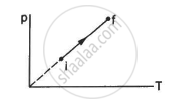• is positive

• is negative

• is zero

• cannot be obtained from this information

MCQ | Q 8 | Page 61

Consider two processes on a system as shown in figure.

The volumes in the initial states are the same in the two processes and the volumes in the final states are also the same. Let ∆W1 and ∆W2 be the work done by the system in the processes A and B respectively.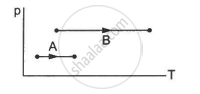• ∆W1 > ∆W2

• ∆W1 = ∆W2

• ∆W1 < ∆W2

• Nothing can be said about the relation between ∆W1 and ∆W2

MCQ | Q 9 | Page 61

A gas is contained in a metallic cylinder fitted with a piston. The piston is suddenly moved in to compress the gas and is maintained at this position. As time passes the pressure of the gas in the cylinder ______________ .

• increases

• decreases

• remains constant

• increases or decreases depending on the nature of the gas

#### Chapter 4: Laws of Thermodynamics Exercise MCQ solutions [Pages 61 - 62]

MCQ | Q 1 | Page 61

The pressure p and volume V of an ideal gas both increase in a process.

(a) Such a process is not possible.

(b) The work done by the system is positive.

(c) The temperature of the system must increase.

(d) Heat supplied to the gas is equal to the change in internal energy.

MCQ | Q 2 | Page 61

In a process on a system, the initial pressure and volume are equal to the final pressure and volume.

(a) The initial temperature must be equal to the final temperature.

(b) The initial internal energy must be equal to the final internal energy.

(c) The net heat given to the system in the process must be zero.

(d) The net work done by the system in the process must be zero.

MCQ | Q 3 | Page 62

A system can be taken from the initial state p1, V1 to the final state p2, V2 by two different methods. Let ∆Q and ∆W represent the heat given to the system and the work done by the system. Which of the following must be the same in both the methods?

• ∆Q

• ∆W

• ∆Q + ∆W

• ∆Q − ∆W

MCQ | Q 4 | Page 62

Refer to figure. Let ∆U1 and ∆U2 be the change in internal energy in processes A and B respectively, ∆Q be the net heat given to the system in process A + B and ∆W be the net work done by the system in the process A + B.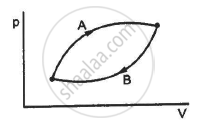(a) ∆U1 + ∆U2 = 0

(b) ∆U1 − ∆U2 = 0

(c) ∆Q − ∆W = 0

(d) ∆Q + ∆W = 0

MCQ | Q 5 | Page 62

The internal energy of an ideal gas decreases by the same amount as the work done by the system.

(a) The process must be adiabatic.

b) The process must be isothermal.

(c) The process must be isobaric.

(d) The temperature must decrease.

#### Chapter 4: Laws of Thermodynamics solutions [Pages 62 - 64]

Q 1 | Page 62

A thermally insulated, closed copper vessel contains water at 15°C. When the vessel is shaken vigorously for 15 minutes, the temperature rises to 17°C. The mass of the vessel is 100 g and that of the water is 200 g. The specific heat capacities of copper and water are 420 J kg−1 K−1 and 4200 J kg−1 K−1 respectively. Neglect any thermal expansion. (a) How much heat is transferred to the liquid-vessel system? (b) How much work has been done on this system? (c) How much is the increase in internal energy of the system?

Q 2 | Page 62

Figure shows a paddle wheel coupled to a mass of 12 kg through fixed frictionless pulleys. The paddle is immersed in a liquid of heat capacity 4200 J K−1 kept in an adiabatic container. Consider a time interval in which the 12 kg block falls slowly through 70 cm. (a) How much heat is given to the liquid? (b) How much work is done on the liquid? (c) Calculate the rise in the temperature of the liquid neglecting the heat capacity of the container and the paddle.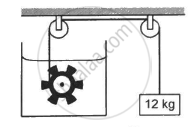Q 2 | Page 62

Figure shows a paddle wheel coupled to a mass of 12 kg through fixed frictionless pulleys. The paddle is immersed in a liquid of heat capacity 4200 J K−1 kept in an adiabatic container. Consider a time interval in which the 12 kg block falls slowly through 70 cm. (a) How much heat is given to the liquid? (b) How much work is done on the liquid? (c) Calculate the rise in the temperature of the liquid neglecting the heat capacity of the container and the paddle.Q 3 | Page 62

A 100 kg lock is started with a speed of 2.0 m s−1 on a long, rough belt kept fixed in a horizontal position. The coefficient of kinetic friction between the block and the belt is 0.20. (a) Calculate the change in the internal energy of the block-belt system as the block comes to a stop on the belt. (b) Consider the situation from a frame of reference moving at 2.0 m s−1 along the initial velocity of the block. As seen from this frame, the block is gently put on a moving belt and in due time the block starts moving with the belt at 2.0 m s−1. calculate the increase in the kinetic energy of the block as it stops slipping  past the belt. (c) Find the work done in this frame by the external force holding the belt.

Q 4 | Page 62

Calculate the change in internal energy of a gas kept in a rigid container when 100 J of heat is supplied to it.

Q 5 | Page 62

The pressure of a gas changes linearly with volume from 10 kPa, 200 cc to 50 kPa, 50 cc. (a) Calculate the work done by the gas. (b) If no heat is supplied or extracted from the gas, what is the change in the internal energy of the gas?

Q 6 | Page 62

An ideal gas is taken from an initial state i to a final state f in such a way that the ratio of the pressure to the absolute temperature remains constant. What will be the work done by the gas?

Q 7 | Page 62

Figure shows three paths through which a gas can be taken from the state A to the state B. Calculate the work done by the gas in each of the three paths.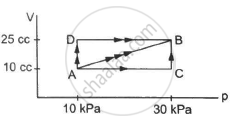Q 8 | Page 62

When a system is taken through the process abc shown in figure, 80 J of heat is absorbed by the system and 30 J of work is done by it. If the system does 10 J of work during the process adc, how much heat flows into it during the process?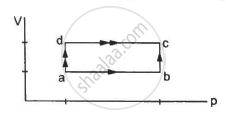Q 9 | Page 63

50 cal of heat should be supplied to take a system from the state A to the state B through the path ACB as shown in figure. Find the quantity of heat to be suppled to take it from A to B via ADB.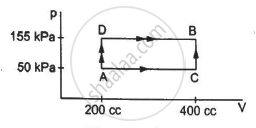Q 10 | Page 63

Calculate the heat absorbed by a system in going through the cyclic process shown in figure.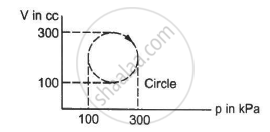Q 11 | Page 63

A gas is taken through a cyclic process ABCA as shown in figure. If 2.4 cal of heat is given in the process, what is the value of J ?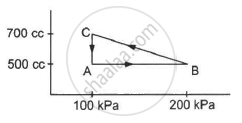Q 12 | Page 63

A substance is taken through the process abc as shown in figure. If the internal energy of the substance increases by 5000 J and a heat of 2625 cal is given to the system, calculate the value of J.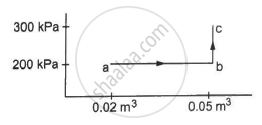Q 13 | Page 63

A gas is taken along the path AB as shown in figure. If 70 cal of heat is extracted from the gas in the process, calculate the change in the internal energy of the system.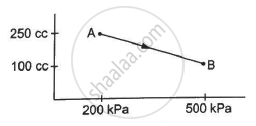Q 14 | Page 63

The internal energy of a gas is given by U = 1.5 pV. It expands from 100 cm3 to 200 cm3against a constant pressure of 1.0 × 105 Pa. Calculate the heat absorbed by the gas in the process.

Q 15 | Page 63

A gas is enclosed in a cylindrical vessel fitted with a frictionless piston. The gas is slowly heated for some time. During the process, 10 J of heat is supplied and the piston is found to move out 10 cm. Find the increase in the internal energy of the gas. The area of cross section of the cylinder = 4 cm2 and the atmospheric pressure = 100 kPa.

Q 16 | Page 63

A gas is initially at a pressure of 100 kPa and its volume is 2.0 m3. Its pressure is kept constant and the volume is changed from 2.0 m3 to 2.5 m3. Its Volume is now kept constant and the pressure is increased from 100 kPa to 200 kPa. The gas is brought back to its initial state, the pressure varying linearly with its volume. (a) Whether the heat is supplied to or extracted from the gas in the complete cycle? (b) How much heat was supplied or extracted?

Q 17 | Page 63

Consider the cyclic process ABCA, shown in figure, performed on a sample of 2.0 mol of an ideal gas. A total of 1200 J of heat is withdrawn from the sample in the process. Find the work done by the gas during the part BC.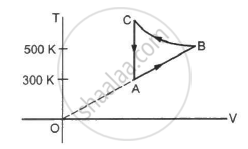Q 18 | Page 63

Figure shows the variation in the internal energy U with the volume V of 2.0 mol of an ideal gas in a cyclic process abcda. The temperatures of the gas at b and c are 500 K and 300 K respectively. Calculate the heat absorbed by the gas during the process.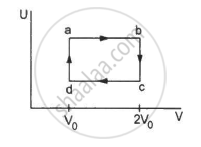Q 19 | Page 63

Find the change in the internal energy of 2 kg of water as it is heated from 0°C to 4°C. The specific heat capacity of water is 4200 J kg−1 K−1 and its densities at 0°C and 4°C are 999.9 kg m−3 and 1000 kg m−3 respectively. Atmospheric pressure = 105 Pa.

Q 20 | Page 63

Calculate the increase in the internal energy of 10 g of water when it is heated from 0°C to 100°C and converted into steam at 100 kPa. The density of steam = 0.6 kg m−3. Specific heat capacity of water = 4200 J kg−1 °C−1 and the latent heat of vaporization of water = 2.25 × 10 6J kg−1.

Q 21 | Page 64

Figure shows a cylindrical tube of volume V with adiabatic walls containing an ideal gas. The internal energy of this ideal gas is given by 1.5 nRT. The tube is divided into two equal parts by a fixed diathermic wall. Initially, the pressure and the temperature are p1, T1 on the left and p2, T2 on the right. The system is left for sufficient time so that the temperature becomes equal on the two sides. (a) How much work has been done by the gas on the left part? (b) Find the final pressures on the two sides. (c) Find the final equilibrium temperature. (d) How much heat has flown from the gas on the right to the gas on the left?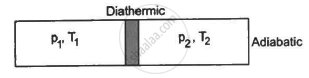Q 22 | Page 64

An adiabatic vessel of total volume V is divided into two equal parts by a conducting separator. The separator is fixed in this position. The part on the left contains one mole of an ideal gas (U = 1.5 nRT) and the part on the right contains two moles of the same gas. Initially, the pressure on each side is p. The system is left for sufficient time so that a steady state is reached. Find (a) the work done by the gas in the left part during the process, (b) the temperature on the two sides in the beginning, (c) the final common temperature reached by the gases, (d) the heat given to the gas in the right part and (e) the increase in the internal energy of the gas in the left part.

## Chapter 4: Laws of Thermodynamics

#### H.C. Verma Concepts of Physics - Vol. 2## H.C. Verma solutions for Class 12 Physics chapter 4 - Laws of Thermodynamics

H.C. Verma solutions for Class 12 Physics chapter 4 (Laws of Thermodynamics) include all questions with solution and detail explanation. This will clear students doubts about any question and improve application skills while preparing for board exams. The detailed, step-by-step solutions will help you understand the concepts better and clear your confusions, if any. Shaalaa.com has the CBSE Concepts of Physics - Vol. 2 solutions in a manner that help students grasp basic concepts better and faster.

Further, we at Shaalaa.com provide such solutions so that students can prepare for written exams. H.C. Verma textbook solutions can be a core help for self-study and acts as a perfect self-help guidance for students.

Concepts covered in Class 12 Physics chapter 4 Laws of Thermodynamics are Isothermal Processes, Adiabatic Processes, Thermal Equilibrium, Zeroth Law of Thermodynamics, Heat, Internal Energy and Work, First Law of Thermodynamics, Specific Heat Capacity, Thermodynamic State Variables and Equation of State, Thermodynamic Processes, Heat Engines, Refrigerators and Heat Pumps, Second Law of Thermodynamics, Reversible and Irreversible Processes, Carnot Engine, Temperature and Heat, Measurement of Temperature, Ideal-gas Equation and Absolute Temperature, Thermal Expansion, Specific Heat Capacity, Calorimetry, Change of State - Latent Heat Capacity, Conduction, Convection, Radiation, Newton’s Law of Cooling, Qualitative Ideas of Blackbody Radiation, Wein'S Displacement Law, Stefan's Law, Anomalous Expansion of Water, Liquids and Gases, Thermal Expansion of Solids, Green House Effect.

Using H.C. Verma Class 12 solutions Laws of Thermodynamics exercise by students are an easy way to prepare for the exams, as they involve solutions arranged chapter-wise also page wise. The questions involved in H.C. Verma Solutions are important questions that can be asked in the final exam. Maximum students of CBSE Class 12 prefer H.C. Verma Textbook Solutions to score more in exam.

Get the free view of chapter 4 Laws of Thermodynamics Class 12 extra questions for Physics and can use Shaalaa.com to keep it handy for your exam preparation

S